# Circle toolbarCircle

Draw a circle from center and radius, diameter, points on the circumference, and circumference length.Circle, Diameter

Draws a circle from points on diameter.Circle, 3Point

Draws a circle from 3 points on circumference.Circle, AroundCurve

Draws a circle around a curve.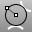Circle, Tangent, Tangent, Radius

Draws a circle tangent to two curves and a radius.Circle, Tangent

Draws a circle tangent to 3 curves.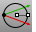Circle, Vertical to CPlane: center, radius

Draws a circle from a center and radius vertical to CPlane.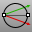Circle, Vertical to CPlane: diameter

Draws a circle from points on diameter vertical to CPlane.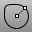Circle, Deformable

Draws a deformable NURBS circle.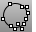Circle, Fit Points

Fits a circle through points.

Rhinoceros 5 © 2010-2015 Robert McNeel & Associates. 17-Sep-2015